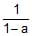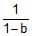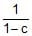JEE  >  Competition Level Test: Straight Line- 4

# Competition Level Test: Straight Line- 4

Test Description

## 27 Questions MCQ Test Mathematics For JEE | Competition Level Test: Straight Line- 4

Competition Level Test: Straight Line- 4 for JEE 2023 is part of Mathematics For JEE preparation. The Competition Level Test: Straight Line- 4 questions and answers have been prepared according to the JEE exam syllabus.The Competition Level Test: Straight Line- 4 MCQs are made for JEE 2023 Exam. Find important definitions, questions, notes, meanings, examples, exercises, MCQs and online tests for Competition Level Test: Straight Line- 4 below.
Solutions of Competition Level Test: Straight Line- 4 questions in English are available as part of our Mathematics For JEE for JEE & Competition Level Test: Straight Line- 4 solutions in Hindi for Mathematics For JEE course. Download more important topics, notes, lectures and mock test series for JEE Exam by signing up for free. Attempt Competition Level Test: Straight Line- 4 | 27 questions in 54 minutes | Mock test for JEE preparation | Free important questions MCQ to study Mathematics For JEE for JEE Exam | Download free PDF with solutions
 1 Crore+ students have signed up on EduRev. Have you?
Competition Level Test: Straight Line- 4 - Question 1

### The co-ordinates of the vertices P, Q, R & S of square PQRS inscribed in the triangle ABC with vertices A(0, 0), B(3, 0) & C(2, 1) given that two of its vertices P, Q are on the side AB are respectively

Detailed Solution for Competition Level Test: Straight Line- 4 - Question 1

Let co-ordinates of P are (x1,0) and side of square is 'a'
∴ Q(x1+a,0)
S(x1, a)
R(x1+a, a)
Now, mAS = mAC
⇒ a/x1 = 1/2
​⇒ x1 = 2a ......(1)
mBR = mBC
⇒ a/(x1+a−3) = −1
⇒ x1+2a−3=0 ......(2)
from (1) & (2) a = 3/4
​& x1 = 3/2
Hence co-ordinates of P, Q, R, & S can be determined.
(3/2,0) (9/4,0) (9/4,3/4) (3/2,3/4)

Competition Level Test: Straight Line- 4 - Question 2

### Points A & B are in the first quadrant ; point 'O' is the origin. If the slope of OA is 1, slope of OB is 7 and OA = OB, then the slope of AB is

Competition Level Test: Straight Line- 4 - Question 3

### If origin and (3, 2) are contained in the same angle of the lines 2x + y – a = 0, x – 3y + a = 0, then 'a' must lie in the interval

Competition Level Test: Straight Line- 4 - Question 4

A(x1, y1), B(x2, y2) and C(x3, y3) are three non-collinear points in cartesian plane. Number of parallelograms that can be drawn with these three points as vertices are

Competition Level Test: Straight Line- 4 - Question 5

Three vertices of triangle ABC are A(-1, 11), B(–9, –8) and C(15, -2). The equation of angle bisector of angle A is

Competition Level Test: Straight Line- 4 - Question 6

If line y – x + 2 = 0 is shifted parallel to itself towards the positive direction of the x-axis by a perpendicular distance of 3√2units, then the equation of the new line is

Detailed Solution for Competition Level Test: Straight Line- 4 - Question 6

Given line:− y−x+2=0
line parallel to y−x+2=0 is
y−x+c=0
distance between these two lines=3√2
Distance, d = |c2−c1|/(√a2+b2)
so, 3√2 = |c−2|/√(−1)2 + (1)2
3√2 = |c−2|/√2
⇒|c−2| = 6
so, c−2 = 6               (since line is shifted towards positive x−axis)
c=8
Equation of new line is y−x+8=0

Competition Level Test: Straight Line- 4 - Question 7

If the axes are rotated through an angle of 30º in the anti-clockwise direction, the coordinates of point (4, –2√3) with respect to new axes are

Competition Level Test: Straight Line- 4 - Question 8

Keeping the origin constant axes are rotated at an angle 30º in clockwise direction then new coordinate of (2, 1) with respect to old axes is

Competition Level Test: Straight Line- 4 - Question 9

If one diagonal of a square is along the line x = 2y and one of its vertex is (3, 0), then its sides through this vertex are given by the equations

Competition Level Test: Straight Line- 4 - Question 10

The equation 2x2 + 4xy – py2 + 4x + qy + 1 = 0 will represent two mutually perpendicular straight lines, if

Competition Level Test: Straight Line- 4 - Question 11

One of the diameter of the circle circumscribing the rectangle ABCD is 4y = x + 7. If A and B are the points (–3, 4) and (5, 4) respectively then the area of rectangle is equal to

Detailed Solution for Competition Level Test: Straight Line- 4 - Question 11

Midpoint of AB=(5−3/2 , 4+4/2) = (1,4)
E≡(1,4)
y-coordinate of A, E, B are same
⇒AB∣∣x-axis
⇒ x-coordinate of =x-coordinate of E centre O
⇒Ox = 1
Now, 4y=x+7 is a diameter hence, it necessarily passes through the centre of the circle O
⇒4y=x+7(x=1)
⇒4y=8
⇒y=2
⇒O≡(1,2)
Now, OE=OF
∵OE=4−2=2
⇒OF=2
⇒EF=2+2=4
AB=5+3=8
EF=BC=4
⇒ Area of rectangle ABCD=L×B=AB×BC=8×4=32

Competition Level Test: Straight Line- 4 - Question 12

The co-ordinates of a point P on the line 2x–y+5=0 such that |PA – PB| is maximum where A is (4, –2) and B is (2, –4) will be

Competition Level Test: Straight Line- 4 - Question 13

The line x + y = p meets the axis of x and y at A and B respectively. A triangle APQ is inscribed in the triangle OAB, O being the origin, with right angle at Q, P and Q lie respectively on OB and AB. If the area of the triangle APQ is 3/8th of the area of the triangle OAB, then AQ/BQ is equal to

Competition Level Test: Straight Line- 4 - Question 14

Lines, L1 : x + √3y = 2, and L2 : ax + by = 1, meet at P and enclose an angle of 45º between them. Line L3 : y = , also passes through P then

Competition Level Test: Straight Line- 4 - Question 15

A triangle is formed by the lines 2x – 3y – 6 = 0 ; 3x – y + 3 = 0 and 3x + 4y – 12 = 0. If the points P(a, 0) and Q(0, b) always lie on or inside the DABC, then

Competition Level Test: Straight Line- 4 - Question 16

The line x + 3y – 2 = 0 bisects the angle between a pair of straight lines of which one has equation x – 7y + 5 = 0. The equation of the other line is

Competition Level Test: Straight Line- 4 - Question 17

A ray of light passing through the point A(1, 2) is reflected at a point B on the x-axis and then passes through (5, 3). Then the equation of AB is

Competition Level Test: Straight Line- 4 - Question 18

Let the algebraic sum of the perpendicular distances from the point (3, 0), (0, 3) & (2, 2) to a variable straight line be zero, then the line passes through a fixed point whose co-ordinates are

Competition Level Test: Straight Line- 4 - Question 19

The pair of straight lines x2 – 4xy + y2 = 0 together with the line x + y + = 0 form a triangle which is

Competition Level Test: Straight Line- 4 - Question 20

Let Aº (3, 2) and Bº (5, 1). ABP is an equilateral triangle is constructed on the side of AB remote from the origin then the orthocentre of triangle ABP is

Competition Level Test: Straight Line- 4 - Question 21

The line PQ whose equation is x – y = 2 cuts the x-axis at P and Q is (4, 2). The line PQ is rotated about P through 45º in the anticlockwise direction. The equation of the line PQ in the new position is

Competition Level Test: Straight Line- 4 - Question 22

Distance between two lines represented by the line pair, x2 – 4xy + 4y2 + x – 2y – 6 = 0 is

Competition Level Test: Straight Line- 4 - Question 23

The circumcentre of the triangle formed by the lines, xy + 2x + 2y + 4 = 0 and x + y + 2 = 0 is

Competition Level Test: Straight Line- 4 - Question 24

Area of the rhombus bounded by the four lines, ax ± by ± c = 0 is

Competition Level Test: Straight Line- 4 - Question 25

If the lines ax + y + 1 = 0, x + by + 1 = 0 & x + y + c = 0 where a, b & c are distinct real numbers different from 1 are concurrent, then the value of++equals

Competition Level Test: Straight Line- 4 - Question 26

The area enclosed by 2 | x | + 3| y | < 6 is

Competition Level Test: Straight Line- 4 - Question 27

The point (4, 1) undergoes the following three transformations successively
(i) Reflection about the line y = x
(ii) Translation through a distance 2 units along the positive direction of x-axis
(iii) Rotation through an angle p/4 about the origin in the counter clockwise direction.
The final position of the points is given by the coordinates

## Mathematics For JEE

130 videos|359 docs|306 tests
 Use Code STAYHOME200 and get INR 200 additional OFF Use Coupon Code
Information about Competition Level Test: Straight Line- 4 Page
In this test you can find the Exam questions for Competition Level Test: Straight Line- 4 solved & explained in the simplest way possible. Besides giving Questions and answers for Competition Level Test: Straight Line- 4, EduRev gives you an ample number of Online tests for practice

## Mathematics For JEE

130 videos|359 docs|306 tests